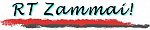Sitemap | Coverstory | Photos | Railways z. | Bridges z. | Seeds
\$B8=:_\$N%Z!<%8(B :
 \$B%H%C%W(B >> \$B%P!<%_%s%,%`JX\$j(B >> 2001\$BG/(B >> 7-9\$B7n(B >> \$B%G%8%+%aJX\$j(B \$BBh(B2\$B2sJd0d(B
You are now in :
 Top >> From Birmingham >> Yr. 2001 >> 3rd Q. >> Digital Camera Report No. 2 Addendum

\$B%P!<%_%s%,%`!&%G%8%+%aJX\$j(B
Digital Camera Report from Birmingham

# \$BBh(B2\$B2sJd0d(B \$B!V(BRailtrack \$BGKC>\$H\$3\$N(B1\$B=54V\$N1Q9qFb\$NJsF;!W(BNo. 2 Addendum: "The bankruptcy of Railtrack and a week's mass media reports"

\$BBh(B2\$B2s869F\$r=q\$\$\$F\$+\$iH>7n\$N\$&\$A\$K!"(B Railtrack \$BGKC>\$H\$\$\$&%K%e!<%9\$,Ht\$S9~\$s\$G\$-\$?!#(B \$B\$=\$3\$G!"(B \$BDy@ZD>A0\$@\$,\$3\$l\$K\$^\$D\$o\$k:G6a(B1\$B=54V\$NJsF;\$r4JC1\$K\$^\$H\$a\$F5-\$9!#(B \$B\$?\$@!"(B \$B4pK\\$,@lLg30\$N7P1DLdBj\$J\$&\$(!"(B \$B\$b\$H\$NL11D2=%9%-!<%`\$9\$i\$-\$A\$s\$HM}2r\$G\$-\$F\$\$\$J\$\$\$N\$G!"(B \$B8m\$j\$J\$I\$N2DG=@-\$,9b\$\$\$3\$H\$O\$*CG\$j\$7\$F\$*\$-\$?\$\$!#(B

Railtrack \$B\$N\$h\$&\$J2ql\$7\$?(B C. Wolmar \$B;a\$O!"(B \$B=;ED@5Fs;a\$NCx=q(B "Success Story"\$B!JK.BjITL@!K\$N8@MU\$r0z\$-\$J\$,\$i!"(B \$B\$3\$NOHAH\$_\$K\$O:G=i\$+\$iLdBj\$,\$"\$C\$?!"(B \$B\$H=R\$Y\$k!#(B \$B!VMx1W\$r>e\$2\$k\$?\$a\$K\$O!"(B \$B@~O);HMQ\$NBP2A\$r>e\$2\$k\$+!"(B \$B\$5\$b\$J\$/\$PJ]J}\$rF1;~\$K\$d\$C\$F!"(B \$BGKLGE*\$J7k2L\$r>7\$\$\$?!W(B

\$B\$=\$NE57?Nc\$,!"(B Southall (1997\$BG/(B9\$B7n!"(B7\$B?M;`K4(B)\$B!"(B Ladbroke Grove (1999\$BG/(B10\$B7n!"(B31\$B?M;`K4(B)\$B!"(B Hatfield \$B;v8N(B(2000\$BG/(B10\$B7n!"(B4\$B?M;`K4(B)\$B\$N(B3\$BBg;v8N\$G!"(B \$BE4F;\$N0BA4@-\$KBg\$-\$J5?LdId\$,\$D\$/\$3\$H\$H\$J\$C\$?!#(B

\$BLdBj\$O\$=\$l\$@\$1\$K\$H\$I\$^\$i\$J\$\$!#(B \$BNse\$7!"(B \$B?6;Re!"(B \$B\$3\$l\$i%W%m%8%'%/%H\$N\$?\$a@/I\\$+\$i\$N;q6b\$b \$B\$3\$s\$J2qe8x6b\$r\$D\$.9~\$`\$N\$OL5BL!"(B \$B\$HH=CG\$7\$?(B Stephen Byers \$B1?M"Bg?C\$,!"(B \$BL11D2=\$NOHAH\$_\$rDj\$a\$?(B Railways Act 1993 \$B\$NGKC>=hM}>r9`\$K=>\$C\$F6/0z\$J9SNE<#\$KBG\$C\$F=P\$?\$H\$\$\$&\$N\$,!"(B 10\$B7n(B6\$B!A(B7\$BF|\$N=5Kv\$N=PMh;v\$@\$C\$?\$o\$1\$@!#(B

\$B\$@\$,!"(B \$B:#8e\$I\$N\$h\$&\$K:FJT\$,9T\$o\$l\$k\$+\$ON.F0E*\$@!#(B Byers \$B;a\$O(B Railtrack \$B\$N\$+\$o\$j\$K3tpJs\$b\$"\$j!"(B Byers \$B;a\$b(B \$B!V2f!9\$N9M\$(\$k0F\$HF1Ey0J>e\$N\$b\$N!W(B \$B\$G\$"\$k\$J\$i9MN8\$NM>CO\$,\$"\$k\$H\$7\$F\$\$\$k!#(B

\$B@/I\\$O:#8e(B10\$BG/\$G(B300\$B2/%]%s%I\$rE4F;\$K;Y=P\$9\$k\$H\$\$\$C\$F\$O\$\$\$k\$,!"(B West Coast Main Line \$B2~NI\$r\$O\$8\$a\$H\$7\$F!"(B \$BE4F;%W%m%8%'%/%H\$,:#8e=gD4\$K?J\$`\$H9M\$(\$k8~\$-\$O>/\$J\$\$!#(B

\$B8=:_\$N(B Railtrack \$B\$O(B Ernst & Young \$B\$H\$\$\$&7P1D%3%s%5%k%?%s%H\$N(B administration \$B\$N\$b\$H\$GGKC>=hM}\$KF~\$C\$?!#(B \$BNsD>A0\$N6bMKF|\$N3t2A=*CM\$O(B280\$B%Z%s%9\$H9b\$/!"(B \$BDL>o\$NGKC>4k6H\$H\$OMMAj\$,0[\$J\$k!#(B \$B\$=\$N8e!"(B \$BB?>/\$OJd=~\$,F@\$i\$l\$k\$3\$H\$K\$J\$C\$?\$b\$N\$N!"(B \$B\$3\$N\$h\$&\$J6/0z\$JGKC>=hM}\$K\$h\$C\$F:#8e\$N(B PPP (public-private partnerships) \$B%W%m%8%'%/%H\$K\$*\$\$\$F!"(B \$BL14V;q6b\$ND4C#%3%9%H\$N>e>:\$J\$I\$N0-1F6A\$,7|G0\$5\$l\$F\$\$\$k!#(B

\$B\$^\$?!"(B \$B%m%s%I%sCO2yEO\$7!"(B \$BNse9qM-2=\$5\$l\$kJ}8~\$G\$"\$k\$N\$K%m%s%I%s\$,(B \$B!V5U!W(B \$B\$r\$d\$k\$N\$OIT<+A3!"(B \$B\$H\$\$\$&;XE&\$b\$G\$F\$-\$F\$\$\$k!#(B

Byers \$B;a\$NJsF;BP:v%"%I%P%\$%6\$,!"(B 9\$B7n(B11\$BF|\$N%F%mKVH/\$NLs(B1\$B;~4V8e\$K(B \$B!V\$^\$:\$\$\$3\$H\$rKd\$b\$l\$5\$;\$k(B (bury) \$B\$K\$O@d9%\$NF|\$@!W(B \$B\$H\$\$\$&EE;R%a!<%k\$rAw?.\$7\$?\$3\$H\$bK=O*\$5\$l\$k\$J\$I!"(B \$B;vBV\$O@/<#LdBj2=\$NMMAj\$b\$_\$;\$F\$\$\$k!#(B

\$B\$b\$A\$m\$s!"(B \$BMxMQ

1. \$B\$3\$l\$GNs (Will this make the trains run on time?)
2. \$BB?J,\$@\$a\$@\$m\$&\$M!#(B
(Probably not.)

\$B9bLZ(B \$BN<(B / TAKAGI, Ryo webmaster@takagi-ryo.ac